## Introduction

The SCION Instruments Single Quad (SQ) GC-MS is known for its exceptional performance, the SQ models are capable of handling a wide variety of GC-MS SQ applications. The design is lens free to reduce the loss of ions and increase detector sensitivity. In addition,  the heated optics and an Extended Dynamic Range (EDR) detector enable the SCION SQ to deliver accurate quantification and identification. The exceptional design of this device results in high sensitivity and this results in a low IDL.

Figure 1 shows the SCION 8300 and 8500 GC with  8700 SQ-MS in combination with the 8400 pro autosampler.

Figure 1: SCION Instruments 8300 and 8500 GC with 8700 SQ-MS and a 8400 pro autosampler.

## Instrument Detection Limit (IDL)

The instrument detection limit  (IDL) of  the mass Spectrometer (MS), is defined as the lowest concentration (of an analyte) that can be statistically distinguished from the noise level. The IDL can be calculated using different methods.

One of the methods uses the relation between the standard deviation (STD) of the measured area and the statistical confidence factor t. The calculation can be done using the one sided student t-distribution when number of the measurements is below 30.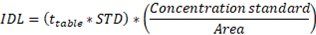Another method uses the signal to noise ratio (SNR). SNR is a measure of the IDL using the  root mean square (RMS) which is the standard deviation of the noise.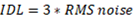The IDL is a concept derived from the limit of detection (LOD) but these terms should not be confused. The LOD is the smallest quantity of analyte that is significantly different from the blank. It can be used for qualitative analysis, but not for quantitative analysis. It is often determined on the basis of three times the noise level. To simplify the LOD is method specific while the IDL is practically a set test for sensitivity.

## Experimentation

The SQ-MS can be implemented on both the 8300-GC as well as on the 8500-GC platform. The Scion 8500-GC analyser used for the determination of the IDL was equipped with an 8400 pro autosampler.

For all experiments 200 fg/µl OFN in iso-octane was used. The IDL value will be determined on multiple SQ-MS systems.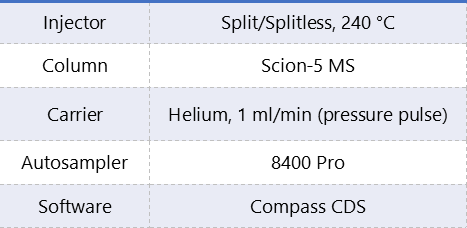Table 1: Analytical conditions 8×00-GC  analyser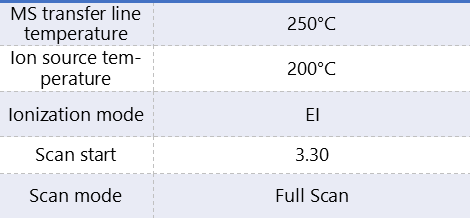Table 2: Analytical conditions Scion SQ GC-MS.

## Results

For the first IDL calculation method a series of eight injections was used. When looking in the t-table it shows a value of 2.9978 (n=7, 99% confidence).

For the eight 200 fg measurements of system 1 the mean value of the area is 8647 with a STD of 455. This gives an IDL of (2.9978*455)/ (200*8647)= 31.6 fg.

System 2 has a mean value of 9235 with a STD of 384. This gives an IDL of (2.9978*384)/ (200*9235)= 24.9 fg.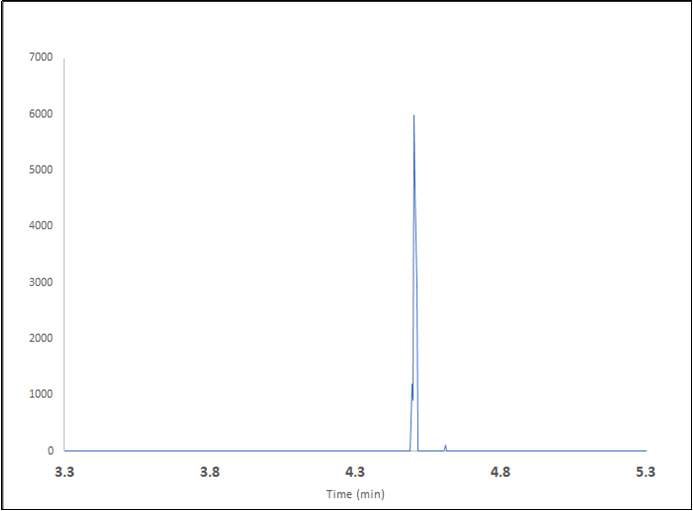Figure 2: EI full scan extracted ion chromatogram of m/z 272 from 200 fg OFN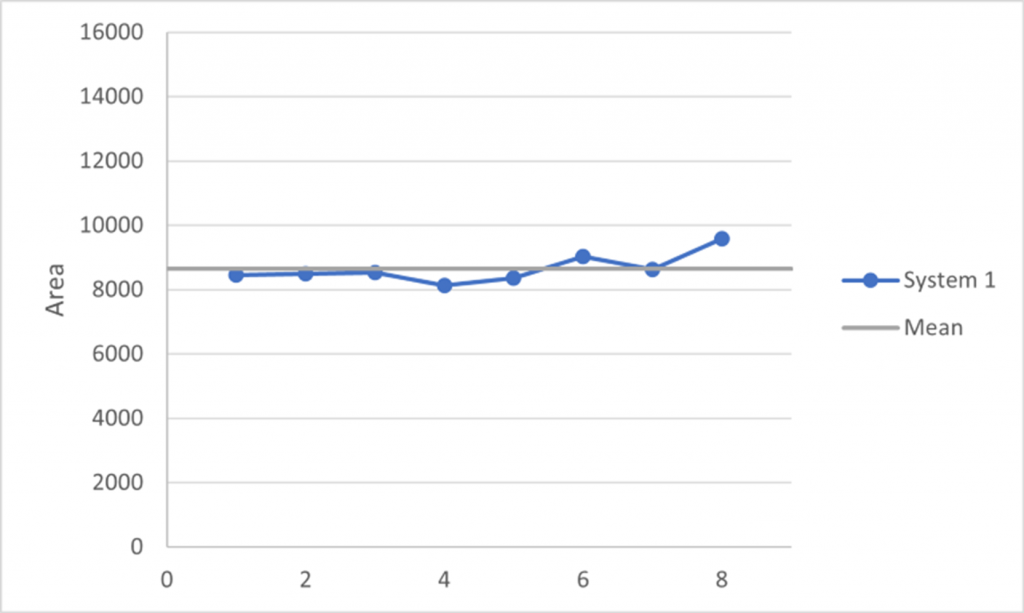Figure 3: Peak area response for eight measurements of 200 fg OFN from system 1.

The Average IDL of the two systems is 28.3 fg, this means that a concentration equal or greater than 28.3 is detectable with a 99% probability.

The other method using three times the noise as a calculation for the IDL shows significant lower results than the other method. In table 3 it is shown that the IDL’s  for both systems are lower than 1 fg for EI source SQ’s.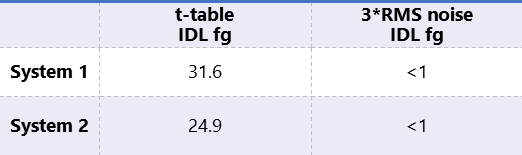Table 3:  Average IDL concentrations in fg using the two different calculations.

## Conclusion

The Scion 8500-GC analyser equipped with a split/spitless injector, Scion Instruments column and a Scion Instruments SQ-MS is perfectly capable for analysis on ultra-trace level.

The level of IDL’s achieved for both calculation methods are excellent and opens up new possibilities for applications in different field of analysis.

The Scion SQ-MS is available in a select (EI with unheated quad), prime (EI with heated quad) and premium model. (EI/CI with heated quad)

All test were done on the select base model.